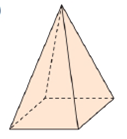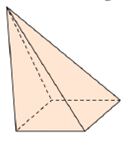Chapter 9.2, Problem 3E### Elementary Geometry for College St...

6th Edition
Daniel C. Alexander + 1 other
ISBN: 9781285195698

#### Solutions

Chapter
Section### Elementary Geometry for College St...

6th Edition
Daniel C. Alexander + 1 other
ISBN: 9781285195698
Textbook Problem
1 views

# In Exercises 1 to 4, name the solid that is shown. Answers are based on Sections 9.1 and 9.2.a)Lateral faces are congruent; base is a square.b)Base is a square.

To determine

(a)

To name:

The solid in the given figure.

Explanation

Given:

The figure given below

and lateral faces are congruent; base is a square.

Properties Used:

A pyramid is made by connecting a base to an apex. The base is flat with straight edges, no curves, hence, a polygon and all other faces are triangles. The altitude/height of the pyramid is the line segment from the vertex perpendicular to the plane of the base.

If the apex is directly above the center of the base, then it is a Right Pyramid. If the apex is not directly above the center of the base, then it is an Oblique Pyramid.

A regular pyramid is a pyramid whose base is a regular polygon and whose lateral edges are all congruent...

To determine

(b)

To name:

The solid in the given figure.

### Still sussing out bartleby?

Check out a sample textbook solution.

See a sample solution

#### The Solution to Your Study Problems

Bartleby provides explanations to thousands of textbook problems written by our experts, many with advanced degrees!

Get Started

#### Find more solutions based on key concepts